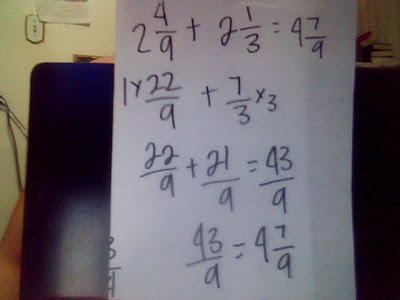### Tracy's Last Fraction Post

Monday, May 11, 2009
1st : Find a common denominator.
2nd : Add the numerator to a numerater.
3rd : Add the denominator to a denominator.
Last : Simplify it. (only if possible)Subtracting Fractions:
1st : Find a common denominator.
2nd : Subtract the numerator to a numerator.
3rd : Subtract the denominator to a denominator.
Last : Simplify it. (only if possible)

1st : Convert the mixed numbers into improper fractions.
2nd : Fina a common denominator.
3rd : Add the numerator to a numerator.
4th : Add the denominator to a denominator.
5th : Convert the answer into a mixed number.
Last : Simplify it. (only if possible)Multiplying Fractions:
1st : Multiply the numerator to a numerator.
2nd : Multiply denominator to a denominator.
Last : Simplify it. (only if possible)

Multiplying Mixed Numbers:
1st : Convert any of the mixed numbers into improper fractions.
2nd : Multiply the numerator to a numerator.
3rd : Multiply the denominator with a denominator.
4th : Convert the answer into a mixed number.
Last : Simplify it. (only if possible)

Dividing Fractions:
1st : Find the reciprical. (one of the fractions upside down)
2nd : Multiply the numerator to a numerator.
3rd : Multiply the denominator to a denominator.
4th : Convert the fraction into a mixed fraction.
Last : Simplify it. (only if possible)
Dividing Mixed Numbers:
1st : Convert the mixed numbers into improper fractions.
2nd : Find the reciprical. (one of the fractions upside down)
3rd : Multiply the numerator to a numerator.
4th : Multiply the denominator to a denominator.
5th : Convert the answer into a mixed number.
Last : Simplify it. (only if possible)

Problem #2
It takes 2 1/4 scoops of flour to make one cake. How many cakes do 15 schoops of flour make?

Problem #3
One day in WInnipeg with 10 1/2 hours of daylight, it was sunny for 1/3 of that time. For how many hours was it sunny that day?